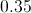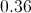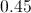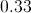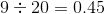SSAT Middle Level Math : How to find the decimal equivalent of a fraction

Example Questions

← Previous 1 3 4

Example Question #1 : How To Find The Decimal Equivalent Of A Fraction

Write the decimal equivalent of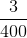.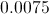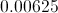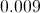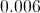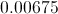Explanation: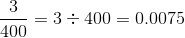Example Question #2 : How To Find The Decimal Equivalent Of A Fraction

Express 0.014 as a fraction in lowest terms.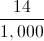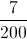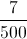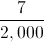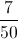Explanation:

The fraction has its last nonzero digit in the thousandths place, so write the number, without the decimal point, over 1,000. Then reduce.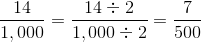Example Question #3 : How To Find The Decimal Equivalent Of A Fraction

Expressas a decimal.Explanation:Example Question #4 : How To Find The Decimal Equivalent Of A Fraction

Which of the following is NOT equal to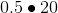?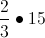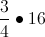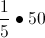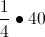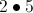Explanation:

All of the answer choices multiply to give you 10 exceptso that is the correct answer.

Example Question #5 : How To Find The Decimal Equivalent Of A Fraction

What fractions equals?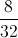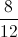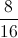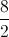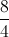Explanation:is equal to.

Thus we are looking for a fraction that reduces to.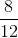reduces to(divide numerator and denominator by 4).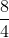reduces to 2 (divide numerator and denominator by 4).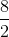reduces to 4 (divide numerator and denominator by 2).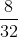reduces(divide numerator and denominator by 4).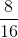is the only one that reduces to(divide numerator and denominator by 8).

Example Question #6 : How To Find The Decimal Equivalent Of A Fraction

Write as a decimal: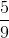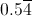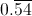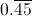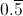Explanation: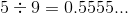That is, the digit 5 will repeat infinitely. This can be written as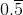.

Example Question #7 : How To Find The Decimal Equivalent Of A Fraction

Write as a decimal: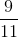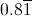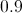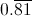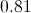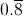Explanation: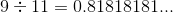That is, the group "81" will repeat infinitely. This quotient can be written as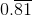.

Example Question #8 : How To Find The Decimal Equivalent Of A Fraction

Rewrite as a decimal: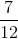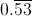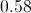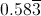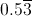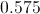Explanation: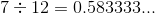That is, after 5 and 8, the digit 3 will repeat infinitely. This can be rewritten as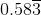.

Example Question #9 : How To Find The Decimal Equivalent Of A Fraction

Write as a decimal: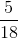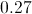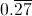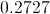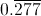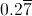Explanation: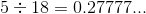That is, after the 2, the digit 7 repeats infinitely. This can be rewritten as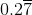.

Example Question #10 : How To Find The Decimal Equivalent Of A Fraction

Express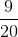as a decimal.